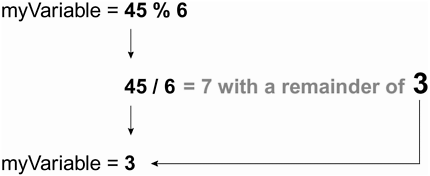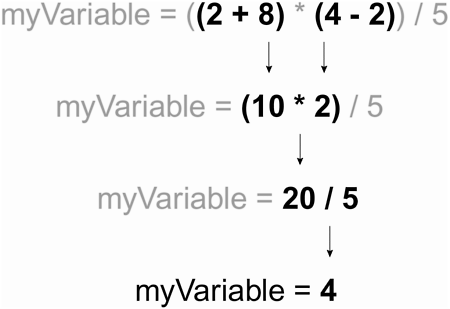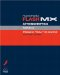# OPERATORS

Operators are the "marks" within an expression that control the way in which the expression's values are evaluated. There are various types of operators; the ones you use will depend on the ways in which you need to manipulate values.

NOTE

In this section, we'll review both arithmetic and string operators. For information about logical and comparison operators, see Lesson 9, Using Conditional Logic.

#### Arithmetic Operators

Even if you're not very familiar with ActionScript, you will be familiar with most of the arithmetic operators. These operators are used in expressions to manipulate numeric values.

• Addition operator (+). Adds two numeric values together. Example: totalCost = productPrice + tax; This adds the two variables to arrive at a final result.

• Increment operator (++). A shorthand method for adding 1 to a value. Example: ++myAge; This increases the value of the myAge variable by 1 the equivalent of myAge = myAge + 1;

• Subtraction operator (-). This operator subtracts two values and can be used in the same way as the addition operator. Example: moneyInWallet = paycheck moneySpent; This subtracts one value from another to return a new number.

• Decrement operator (--). This operator reduces the value of a variable by 1. Example: --bottlesOfBeerOnTheWall; There is now 1 less bottle of beer on the wall.

• Multiplication operator (*). This operator multiplies one numeric value by another. Example: hoursPerWeek = 24 * 7; The number of hours per week is the product of these two numbers being multiplied together.

• Division operator (/). Divides one numeric value by another. Example: hourlyRate = payCheck / hoursBilled; This divides the value of hoursBilled into the value of payCheck .

• Modulo operator (%). Divides the value on the left by the value on the right and returns the value of the remainder. Example: 4 % 2 ; The result of this would be 0 because 4 can be evenly divided by 2, hence there is no remainder. Example: 7 % 3 ; The result of this is 1 because 3 divides into 7 twice with a remainder of 1.#### String Operators

Unlike numbers, which can be manipulated using several different operators, strings can be manipulated by only one operator the Concatenation operator (though they can also be manipulated using various methods of the String object). Although other operators work with strings (namely assignment operators and comparison operators), they cannot be used to directly manipulate a string. (For more on comparison operators, see Lesson 9, Using Conditional Logic.)

• Concatenation operator (+). Concatenation means to link or join two or more things exactly what this operator does with strings of text in ActionScript. The concatenation operator which uses the same symbol as the addition operator (see above) joins two text strings to create a single string. Example: birthDayMessage = "You are " + age + " years old."; If age has a value of 26, then the '+' symbol joins the three parts of the message together to form, "You are 26 years old."

TIP

Unlike some other programming languages, ActionScript does not require you to declare that a variable will hold a string or a number value when you create it. You can sometimes treat a string as a number, and vice versa. With this benefit comes an occasional problem, however: Because the plus (+) symbol is used as both a concatenation operator and an addition operator, Flash must determine whether you're treating a value as a string or a number. This can create some odd issues when loading information from external sources. For example, Flash may see two numbers as strings. PayCheck1 + PayCheck2 may get concatenated instead of added (45 + 65 may end up being "4565" rather then 110). When getting data from an external source or a user-input field, it's sometimes prudent to use the Number() function for example, Number(PayCheck1) + Number(PayCheck2) to ensure that your values are treated as a numeric value (if that's their function).

#### Precedence

Expressions can often include several operators. When this is the case, it's important to understand the order in which parts of the expression are evaluated, or the order of precedence. A value can't be involved in two mathematical operations simultaneously that is, you can't subtract from a value at the same time you're using that value to multiply another number (like the 5 in the expression myNumber = 20 * 5 3 ; one of these evaluations must be completed before the other can begin). Based on the rules of precedence, expressions are evaluated in the following order:

1. Data in parentheses is evaluated before data outside parentheses. For precise control over how an expression is evaluated, you can nest parentheses.

2. Multiplication and division are evaluated before addition or subtraction. Because multiplication and division have equal precedence, evaluation occurs from left to right when both are used (in the absence of parenthesis).

3. Addition and subtraction are evaluated last. Because these operations have equal precedence, evaluation occurs from left to right when both are used (in the absence of parenthesis).

Let's take a look at a few examples:

` myVariable = 5 + 7   3; `

Because addition and subtraction have the same precedence, this expression is simply evaluated from left to right, with myVariable being assigned a value of 9.

` myVariable = 5 + 7 * 3; `

Because multiplication takes precedence over addition, 7 is multiplied by 3, then 5 is added to that result. In the end, myVariable is assigned a value of 26.

` myVariable = (5 + 7) * 3; `

Because data in parentheses takes precedence, 5 is added to 7, then that result is multiplied by 3. In the end, myVariable is assigned a value of 36.

` myVariable = ((2 + 8) * (4   2)) / 5 `

Even though multiplication and division usually take precedence over addition and subtraction, nested parenthesis are used to add 2 to 8, then to subtract 2 from 4. These two results are multiplied, then divided by 5. The result is that myVariable is assigned a value of 4.Macromedia Flash MX ActionScripting: Advanced Training from the Source
ISBN: 0201770229
EAN: 2147483647
Year: 2002
Pages: 161